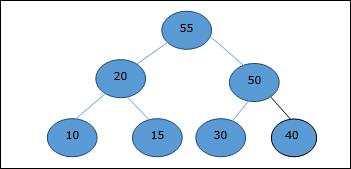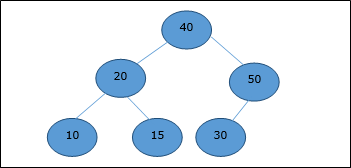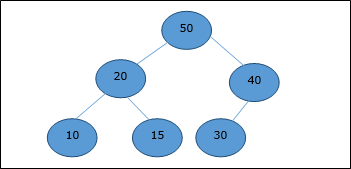• Design and Analysis of Algorithms
• Home

# Design and Analysis Extract Method

Advertisements

Extract method is used to extract the root element of a Heap. Following is the algorithm.

```Algorithm: Heap-Extract-Max (numbers[])
max = numbers
numbers = numbers[heapsize]
heapsize = heapsize – 1
Max-Heapify (numbers[], 1)
return max
```

## Example

Let us consider the same example discussed previously. Now we want to extract an element. This method will return the root element of the heap.After deletion of the root element, the last element will be moved to the root position.Now, Heapify function will be called. After Heapify, the following heap is generated.Advertisements